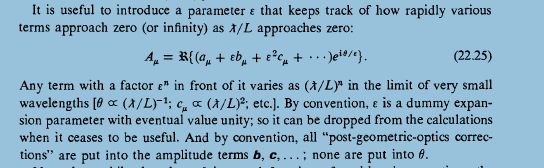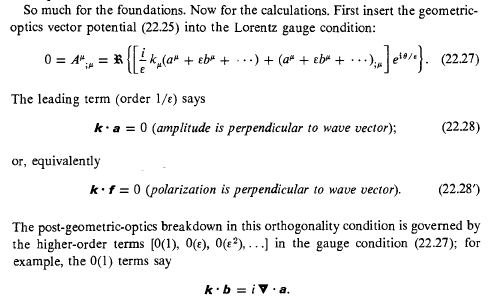# Mysteries of Geometric Optics In MTW Chapter 22

Gold Member
Homework Statement:
I really don't understand what is going on here - can anyone shed any light please?
Relevant Equations:
Contained in attachments
At the start of this section §22.5 (Geometric Optics in curved Spacetime), the amplitude of the vector potential is given as:

A = ##\mathfrak R\{Amplitude \ X \ e^{i\theta}\} ##

The Amplitude is then re=expressed a "two-length-scale" expansion (fine!) but it then is modified further to (22.25) by
introducing a "useful" parameter ε...But this amplitude is now varying at a quite different rate (if ε is anything other than unity) so it isn't equivalent to the original vector potential.

When we get into the calculations, (22.7) is readily derived but I have a problem with the gathering terms O(##\frac {1}{ε}##) or O(ε) etc because, if ε reverts to its eventual value unity thereby recovering the original rate of variation, justification for (22.28) and (22.9) looks a bit thin.Can anyone explain what is going on here and why this is all reasonable?

Regards

TerryW

TSny
Homework Helper
Gold Member
The introduction of the parameter ##\varepsilon## is just a convenient way to "tag" the various terms so that the power of ##\varepsilon## immediately tells you the order of the term in λ/L. A term with ##\varepsilon^2## is second order in λ/L even when ##\varepsilon = 1##. If you want, you could forgo introducing ##\varepsilon##. But, then, you would need to remember that ##a^\mu## is zero order in λ/L, ##b^\mu## is first order, ##c^\mu## is second order, ##\theta## is order -1,etc. When MTW "collect terms of order ##\epsilon^n##", they are just collecting terms of order (λ/L)n, which you could do even if you didn't introduce ##\varepsilon##.

•TerryW
vanhees71
Gold Member
It's not different from the usual eikonal expansion in standard Maxwell theory in flat space. Perhaps it helps to read about this first, e.g., in A. Sommerfeld, Lectures on Theoretical Physics, vol. 4 (optics).

In quantum mechanics a mathematically equivalent procedure is known as WKB approximation. There it's an expansion in powers of ##\hbar##.

The mathematical procedure is known as "singular perturbation theory".

Gold Member
The introduction of the parameter ##\varepsilon## is just a convenient way to "tag" the various terms so that the power of ##\varepsilon## immediately tells you the order of the term in λ/L. A term with ##\varepsilon^2## is second order in λ/L even when ##\varepsilon = 1##. If you want, you could forgo introducing ##\varepsilon##. But, then, you would need to remember that ##a^\mu## is zero order in λ/L, ##b^\mu## is first order, ##c^\mu## is second order, ##\theta## is order -1,etc. When MTW "collect terms of order ##\epsilon^n##", they are just collecting terms of order (λ/L)n, which you could do even if you didn't introduce ##\varepsilon##.
Thanks TSny,

I'd already decided that I didn't need ##\varepsilon## and that I could get to all the results by "remembering" the order of the terms, but your reply is helpful in reassuring me that I wan't missing something crucial.

Cheers

Terry

Gold Member
It's not different from the usual eikonal expansion in standard Maxwell theory in flat space. Perhaps it helps to read about this first, e.g., in A. Sommerfeld, Lectures on Theoretical Physics, vol. 4 (optics).

In quantum mechanics a mathematically equivalent procedure is known as WKB approximation. There it's an expansion in powers of ##\hbar##.

The mathematical procedure is known as "singular perturbation theory".